# Intersection of Two Lines

Intersection of Two Lines
Go back to  'Coordinate Geometry'

In this mini-lesson, we will learn in detail about, How to find the point of intersection of two lines?

We all are familiar with two-dimensional coordinate geometry from the earlier classes.

Primarily, it is a combination of algebra and geometry.

The renowned French philosopher and mathematician, Rene Descartes, published a book, La Géométry in 1637. In this book, he introduced the world with the systematic study of geometry from the use of algebra.

"The intersection of two lines" is an introductory topic under two-dimensional coordinate geometry.

Come, let us learn in detail about how to find the point of intersection of two lines.

In this lesson, we will also learn about intersecting lines examples, the point of intersection formula, and the intersection point calculator.

## Lesson Plan

 1 What Does Intersection of Two Lines Mean? 2 Challenging Questions 3 Solved Examples 4 Important Notes 5 Interactive Questions

## What Does Intersection of Two Lines Mean?

### Intersecting Lines Definition

When two lines share exactly one common point, they are called intersecting lines.

The intersecting lines share a common point. And, this common point that exists on all intersecting lines is called the point of intersection.

Here, lines $$A$$ and $$B$$ intersect at point $$O$$, which is the point of intersection.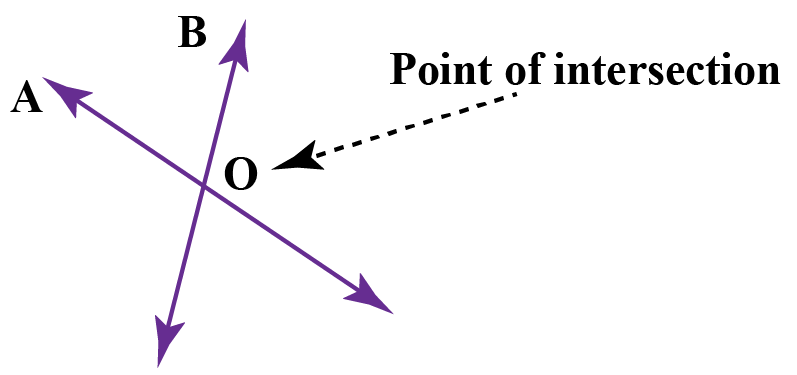### Examples In Real Life

Common examples of intersecting lines in real life include a pair of scissors, a folding chair, a road cross, signboard, etc.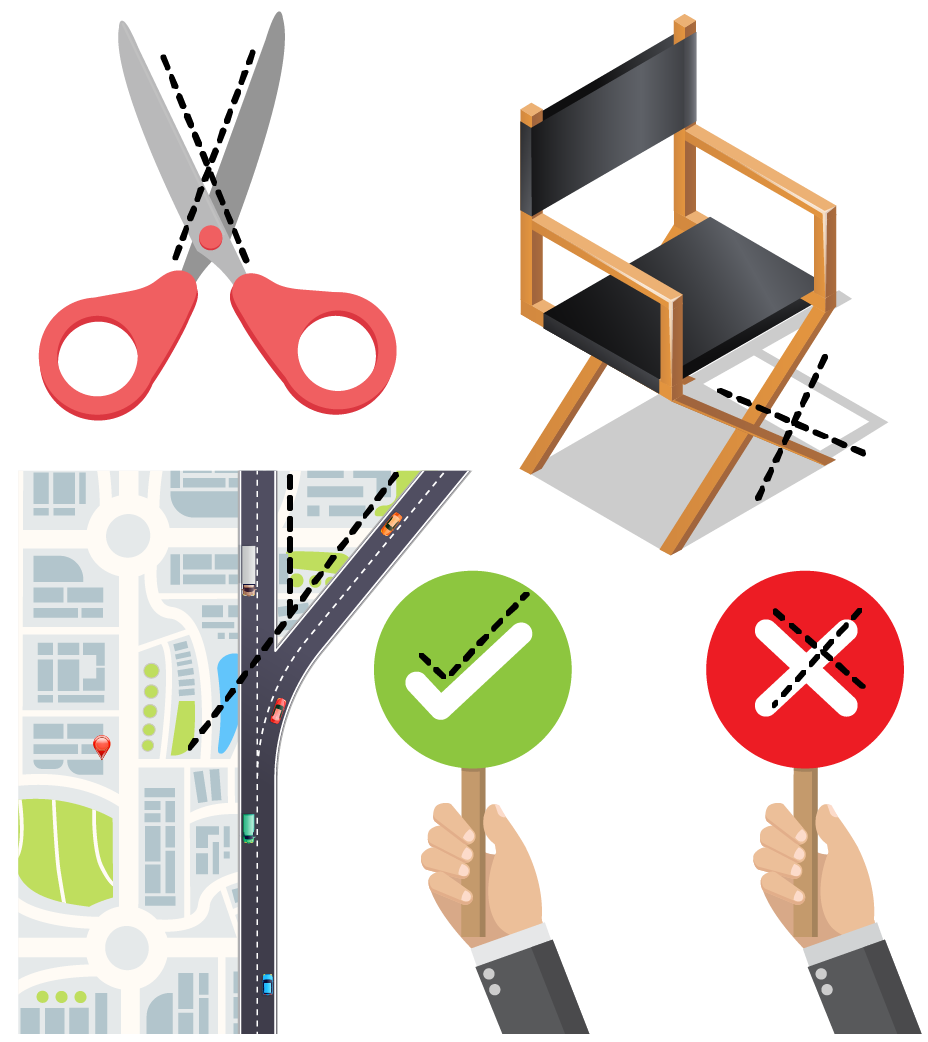Let's consider the following case.

We are given two lines, $${L_1}$$ and $${L_2}$$ , and we are required to find the point of intersection and the angle of intersection.

Evaluating the point of intersection involves solving two simultaneous linear equations.

Let the equations of the two lines be (written in the general form):

$\begin{array}{l}{a_1}x + {b_1}y + {c_1} = 0\\{a_2}x + {b_2}y + {c_2} = 0\end{array}$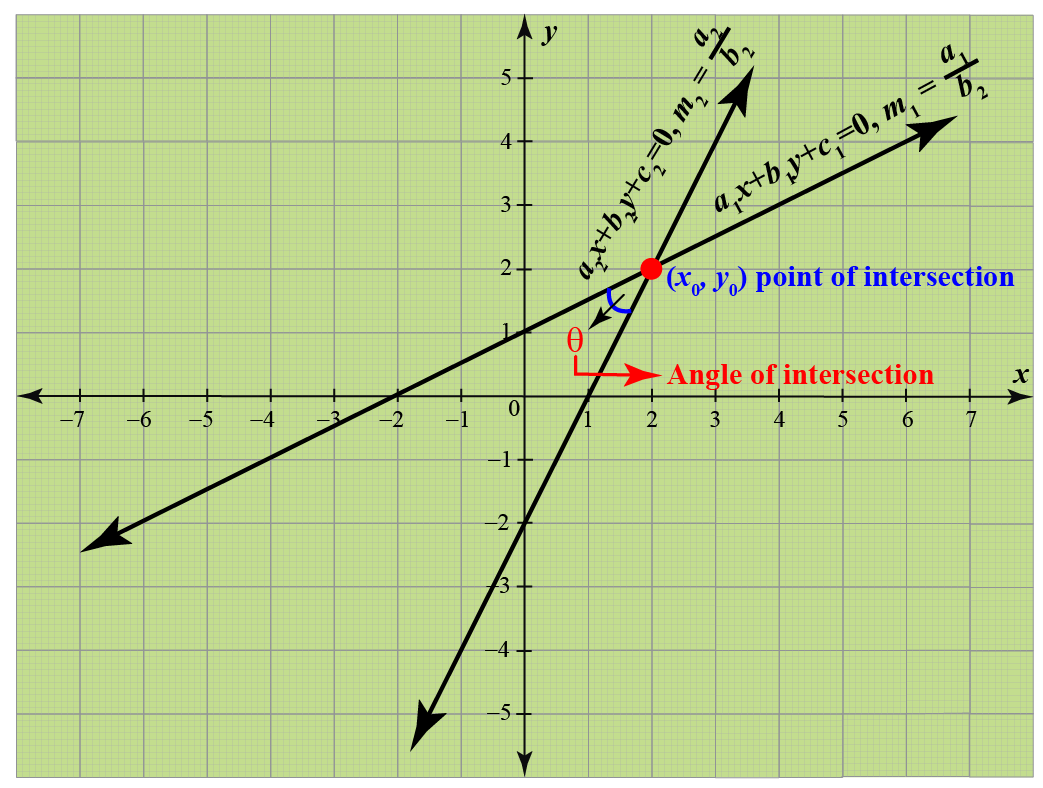Now, let the point of intersection be $$\left( {{x_0},{y_0}} \right)$$. Thus,

$\begin{array}{l}{a_1}{x_0} + {b_1}{y_0} + {c_1} = 0\\{a_2}{x_0} + {b_2}{y_0} + {c_2} = 0\end{array}$

This system can be solved using Cramer’s rule to get:

 $\frac{{{x_0}}}{{{b_1}{c_2} - {b_2}{c_1}}} = \frac{{ - {y_0}}}{{{a_1}{c_2} - {a_2}{c_1}}} = \frac{1}{{{a_1}{b_2} - {a_2}{b_1}}}$

From this relation, we can obtain the point of intersection $$\left( {{x_0},{y_0}} \right)$$ as

 $\left( {{x_0},{y_0}} \right) = \left( {\frac{{{b_1}{c_2} - {b_2}{c_1}}}{{{a_1}{b_2} - {a_2}{b_1}}},\frac{{{c_1}{a_2} - {c_2}{a_1}}}{{{a_1}{b_2} - {a_2}{b_1}}}} \right)$

### Intersection Point Calculator

To obtain the angle of intersection between these two lines, consider the figure shown above.

The equations of the two lines in slope-intercept form are:

\begin{align}&y = \left( { - \frac{{{a_1}}}{{{b_1}}}} \right)x + \left( {\frac{{{c_1}}}{{{b_1}}}} \right) = {m_1}x + {C_1}\\&y = \left( { - \frac{{{a_2}}}{{{b_2}}}} \right)x + \left( {\frac{{{c_2}}}{{{b_2}}}} \right) = {m_2}x + {C_2}\end{align}

Note in the figure above that $$\theta = {\theta _2} - {\theta _1}$$, and thus

 \begin{align}&\tan \theta = \tan \left( {{\theta _2} - {\theta _1}} \right) = \frac{{\tan {\theta _2} - \tan {\theta _1}}}{{1 + \tan {\theta _1}\tan {\theta _2}}}\\&\qquad\qquad\qquad\qquad\;\;= \frac{{{m_2} - {m_1}}}{{1 + {m_1}{m_2}}}\end{align}

Conventionally, we would be interested only in the acute angle between the two lines and thus, we have to have $$\tan \theta$$ as a positive quantity.

So in the expression above, if the expression $$\frac{{{m_2} - {m_1}}}{{1 + {m_1}{m_2}}}$$ turns out to be negative, this would be the tangent of the obtuse angle between the two lines; thus, to get the acute angle between the two lines, we use the magnitude of this expression.

Therefore, the acute angle $$\theta$$ between the two lines is

 $\theta = {\tan ^{ - 1}}\left| {\frac{{{m_2} - {m_1}}}{{1 + {m_1}{m_2}}}} \right|$

From this relation, we can easily deduce the conditions on $${m_1}$$ and $${m_2}$$ such that the two lines $${L_1}$$ and $${L_2}$$ are parallel or perpendicular.

## Conditions for Two Lines to be Parallel or Perpendicular

If the lines are parallel, $$\theta = 0$$ and $${m_1} = {m_2}$$, which is obvious since parallel lines must have the same slope.

For the two lines to be perpendicular lines, $$\theta = \frac{\pi }{2}$$, so that $$\cot \theta = 0$$; this can happen if $$1 + {m_1}{m_2} = 0$$ or $${m_1}{m_2} = - 1$$.

If the lines $${L_1}$$ and $${L_2}$$ are in the general form $$ax + by + c = 0$$, the slope of this line is $$m = - \frac{a}{b}$$.

Condition for Two Lines to be Parallel

Thus, the condition for $${L_1}$$ and $${L_2}$$ to be parallel is:

 ${m_1} = {m_2}\, \Rightarrow \, - \frac{{{a_1}}}{{{b_1}}} = - \frac{{{a_2}}}{{{b_2}}}\, \Rightarrow \,\frac{{{a_1}}}{{{b_1}}} = \frac{{{a_2}}}{{{b_2}}}$

### Example

The line $${L_1}:x - 2y + 1 = 0$$ is parallel to the line $${L_2}:x - 2y - 3 = 0$$ because the slope of both the lines is $$m = \frac{1}{2}$$.

### Condition for Two Lines to be Perpendicular

The condition for $${L_1}$$ and $${L_2}$$ to be perpendicular is:

 \begin{align}&{m_1}{m_2} = - 1\, \Rightarrow \,\left( { - \frac{{{a_1}}}{{{b_1}}}} \right)\left( { - \frac{{{a_2}}}{{{b_2}}}} \right) = - 1\,\\ &\qquad\qquad\;\;\;\; \Rightarrow \,\,{a_1}{a_2} + {b_1}{b_2} = 0\end{align}

### Example

The line $${L_1}:x + y = 1$$ is perpendicular to the line $${L_2}:x - y = 1$$ because the slope of $${L_1}$$ is $$- 1$$ while the slope of $${L_2}$$ is 1.

## Properties of Intersecting Lines

• The intersecting lines (two or more) always meet at a single point.
• The intersecting lines can cross each other at any angle. This angle formed is always greater than $$0^{\circ}$$ and less than $$180^{\circ}$$.
• Two intersecting lines form a pair of vertical angles. The vertical angles are opposite angles with a common vertex  (which is the point of intersection).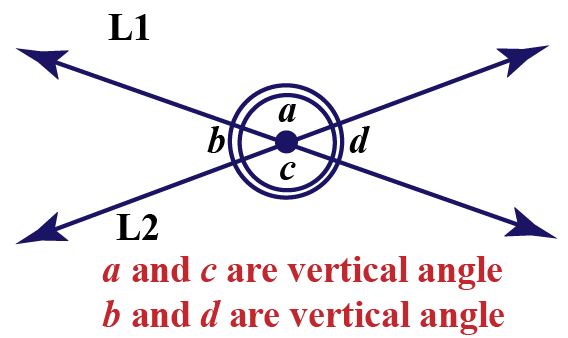Here,$$\angle a$$ and $$\angle c$$ are vertical angles and are equal.

Also, $$\angle b$$ and $$\angle d$$ are vertical angles and equal to each other.

$$\angle a+\angle d$$ = straight angle = $$180^{\circ}$$## Challenging Questions

1. Reduce the following equations into slope-intercept form
(i) $$x+7y=0$$                              (ii) $$3x+2y-12$$
2. If the angle between two lines is $$\frac{\pi}{4}$$ and slope of one the lines is $$\frac{1}{2}$$, find the slope of the other line.

## Solved Examples

 Example 1

Find the point of intersection and the angle of intersection for the following two lines: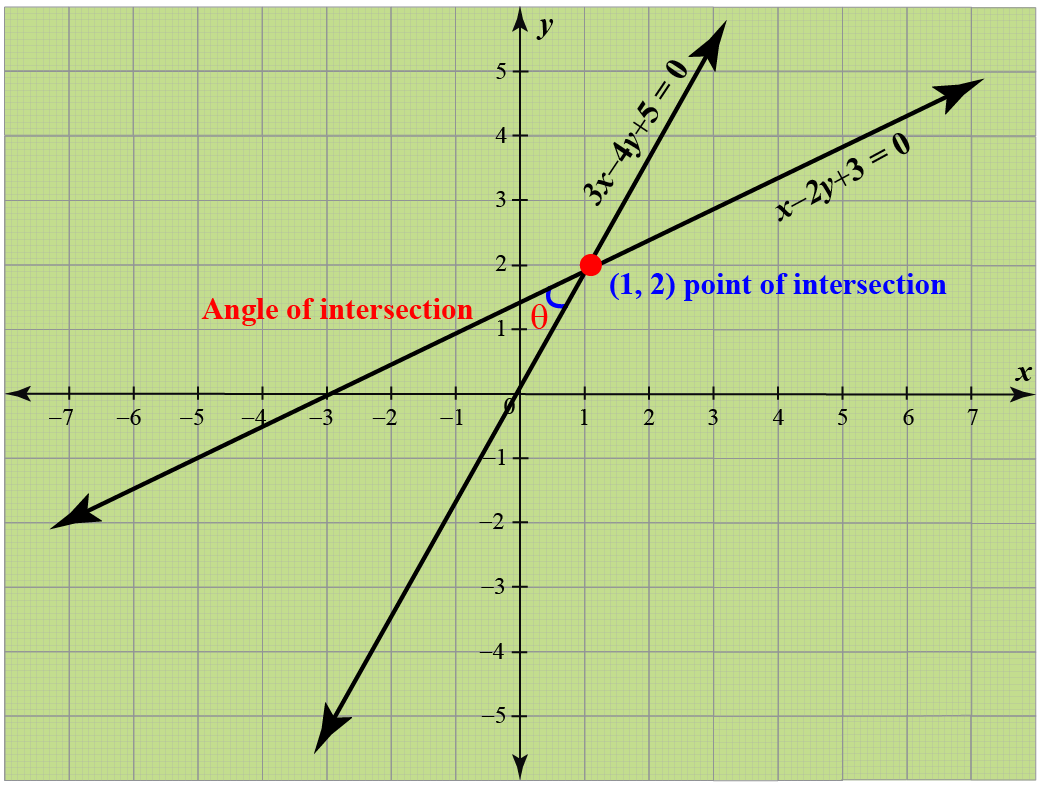$\begin{array}{l}x - 2y + 3 = 0\\3x - 4y + 5 = 0\end{array}$

Solution

We use Cramer’s rule to find the point of intersection:

\begin{align}&\frac{x}{{ - 10 - \left( { - 12} \right)}} = \frac{-y}{{5 -9 }} = \frac{1}{{ - 4 - \left( { - 6} \right)}}\\&\Rightarrow \,\,\,\frac{x}{2} = \frac{y}{4} = \frac{1}{2}\\&\Rightarrow \,\,\,x = 1,\,\,\,y = 2\end{align}
Also, you can check your answer with the help of cuemath's Intersection Point Calculator

Now, the slopes of the two lines are:

${m_1} = \frac{1}{2},\,\,\,{m_2} = \frac{3}{4}$

If $$\theta$$ is the acute angle of intersection between the two lines, we have:

\begin{align}&\tan \theta = \left| {\frac{{{m_2} - {m_1}}}{{1 + {m_1}{m_2}}}} \right| = \left| {\frac{{\frac{3}{4} - \frac{1}{2}}}{{1 + \frac{3}{8}}}} \right| = \frac{2}{{11}}\\&\Rightarrow \,\,\,\theta = {\tan ^{ - 1}}\left( {\frac{2}{{11}}} \right) \approx {10.3^\circ}\end{align}

 $$\therefore$$ Point of intersection is $$(1,2)$$. Angle of intersection is $$\theta=\tan ^{ - 1}\left( {\frac{2}{11}} \right)$$.
 Example 2

Find the equation of a line perpendicular to the line $$x - 2y + 3 = 0$$ and passing through the point$$(1, \;-2)$$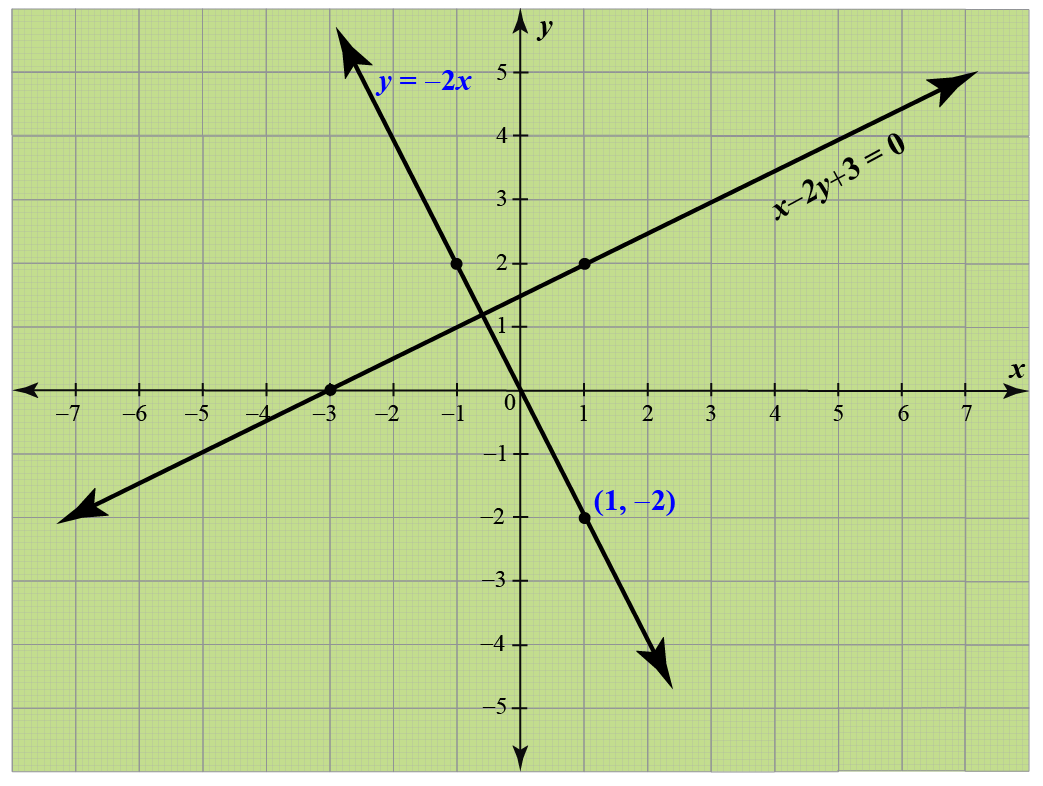Solution

Given line  $$x - 2y + 3 = 0$$ can be written as

${y} = \frac{1}{2}x\,\,\, + \frac{3}{2}$

Slope of the line $$(1)$$ is $${m_1}= \frac{1}{2}$$.

Therefore, slope of the line perpendicular to line $$(1)$$ is

${m_2} = -\frac{1}{{m_1}},\,\,\, = -2$

Equation of a line perpendicular to the line $$x - 2y + 3 = 0$$ and passing through the point$$(1, \;-2)$$ is

$y-(-2) = -2(x-1),\,\,\, \,\,\, \text{or} \,\,\,y= -2x$

Which is the required equation

 $$\therefore$$ Equation of the required line is $$y= -2x$$
Coordinate Geometry
Grade 9 | Questions Set 1
Coordinate Geometry
Coordinate Geometry
Grade 10 | Questions Set 1
Coordinate Geometry